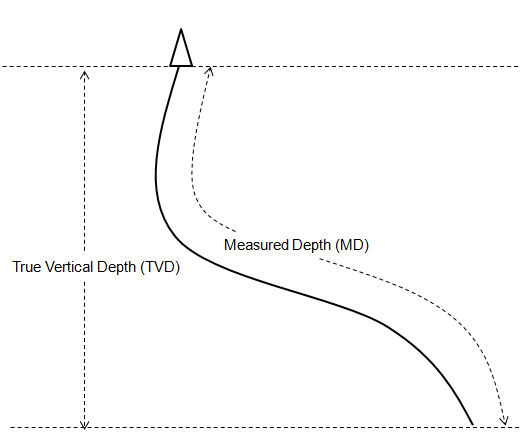# Difference between True Vertical Depth (TVD) and Measured Depth (MD)

I have a question asked regarding what the differences between True Vertical Depth (TVD) and Measured Depth (MD). Today, I will describe both definitions and their importance for calculation in a drilling field.

True Vertical Depth (TVD) is measured vertically from the surface down to a certain target down hole.

Measured Depth (MD) is the total length of the wellbore measured along the actual well path.

See the following illustration for more understanding.True Vertical Depth (TVD) is used for following calculations:

Hydrostatic Pressure Calculation

Hydrostatic Pressure (HP) Decrease When POOH

Formation Temperature Estimation

Drill pipe pulled to lose hydrostatic pressure

Kill Weight Mud Calculation

Formation Pressure from Kick Analysis

Leak Off Test (LOT) Calculation

Formation Integrity Test (FIT) Calculation

Equivalent Circulating Density (ECD)

Surface Pressure During Drill Stem Test

Measured Depth (MD) is used for following calculations:

Annular volume

Drill string and tubular volume

Well control as stokes pump in the drill string and in the annulus, pressure schedule for wait and weight method well control.

Share the joy
Tagged , , , . Bookmark the permalink.Working in the oil field and loving to share knowledge.

### 2 Responses to Difference between True Vertical Depth (TVD) and Measured Depth (MD)

1.Yousef Hasan says:

Hi every one,
what is the equation used to calculate TVD from MD??

Any reference is highly appreciated

•DrillingFormulas.Com says: